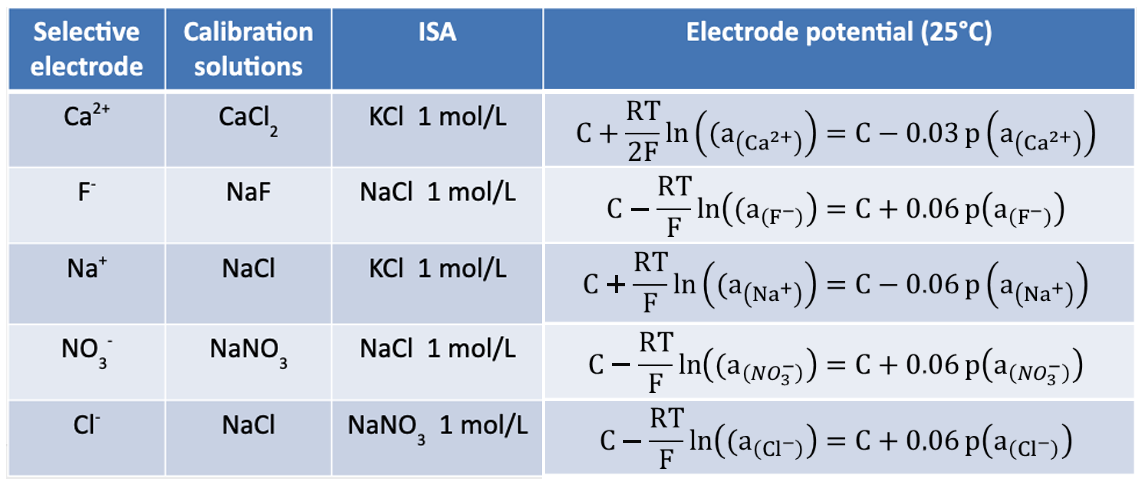## Potentiometry

In this section p corresponds to –log: -log(a) is written as p(a) or pa.

To determine the concentration of the analyte, a calibration curve is determined, using calibration solutions with different concentrations of the analyte (straight line of calibration method). In practice, ΔE = f (p[X]) is plotted with X the analyte ion and ΔE the potential difference (pd) between the selective electrode and a reference electrode. The concentration of X is determined by the measurement of the potential difference (pd) of the sample, called ΔE (sample), and using the calibration straight line.

As with the glass electrode (selective for H+ ions), a potential difference (pd) operates between the inner and outer membranes of the selective electrode. An ion-selective electrode follows the so-called Nikolskii’s law. This law reflects the difference in activity of the ion on each side of the membrane:The electrodes have a sensitivity threshold, corresponding to a value below which the straight line becomes a curve. It is important for the experimenter to be within the linear range of the electrode. This is generally given by the manufacturer, but it can be verified with an experiment.

Moreover, the ionic strength is maintained at a constant level by using a fixed concentration solution (0.1 – 1 mol/L) called ISA (Ionic Strength Adjustor).In further reading, concentration and activity will be considered equivalent.

Next page
Previous page